• 算法收敛性梯度下降法分析算法收敛性 - 精确线搜索exact line search分析算法收敛性 - 非精确线搜索Inexact line search(Amijo Rule) 梯度下降法 dk+1=−∇f(xk)d^{k+1}=-\nabla f\left(x^{k}\right)dk+1=−∇f(xk)f...


算法收敛性
梯度下降法分析算法收敛性 - 精确线搜索exact line search分析算法收敛性 - 非精确线搜索Inexact line search(Amijo Rule)

全部笔记的汇总贴：最优化学习目录

梯度下降法

d

k

+

1

=

−

∇

f

(

x

k

)

d^{k+1}=-\nabla f\left(x^{k}\right)

f

(

x

k

+

1

)

−

P

∗

f

(

x

k

)

−

P

∗

≤

1

−

m

M

\frac{f\left(x^{k+1}\right)-P^{*}}{f\left(x^{k}\right)-P^{*}} \leq 1-\frac{m}{M}

≤

1

−

min

⁡

{

2

m

γ

α

max

⁡

,

2

m

γ

β

M

}

\leq 1-\min \left\{2 m \gamma \alpha_{\max }, \frac{2 m \gamma \beta}{M}\right\}

K

∼

log

⁡

(

f

(

x

k

)

−

P

∗

)

线

性

收

敛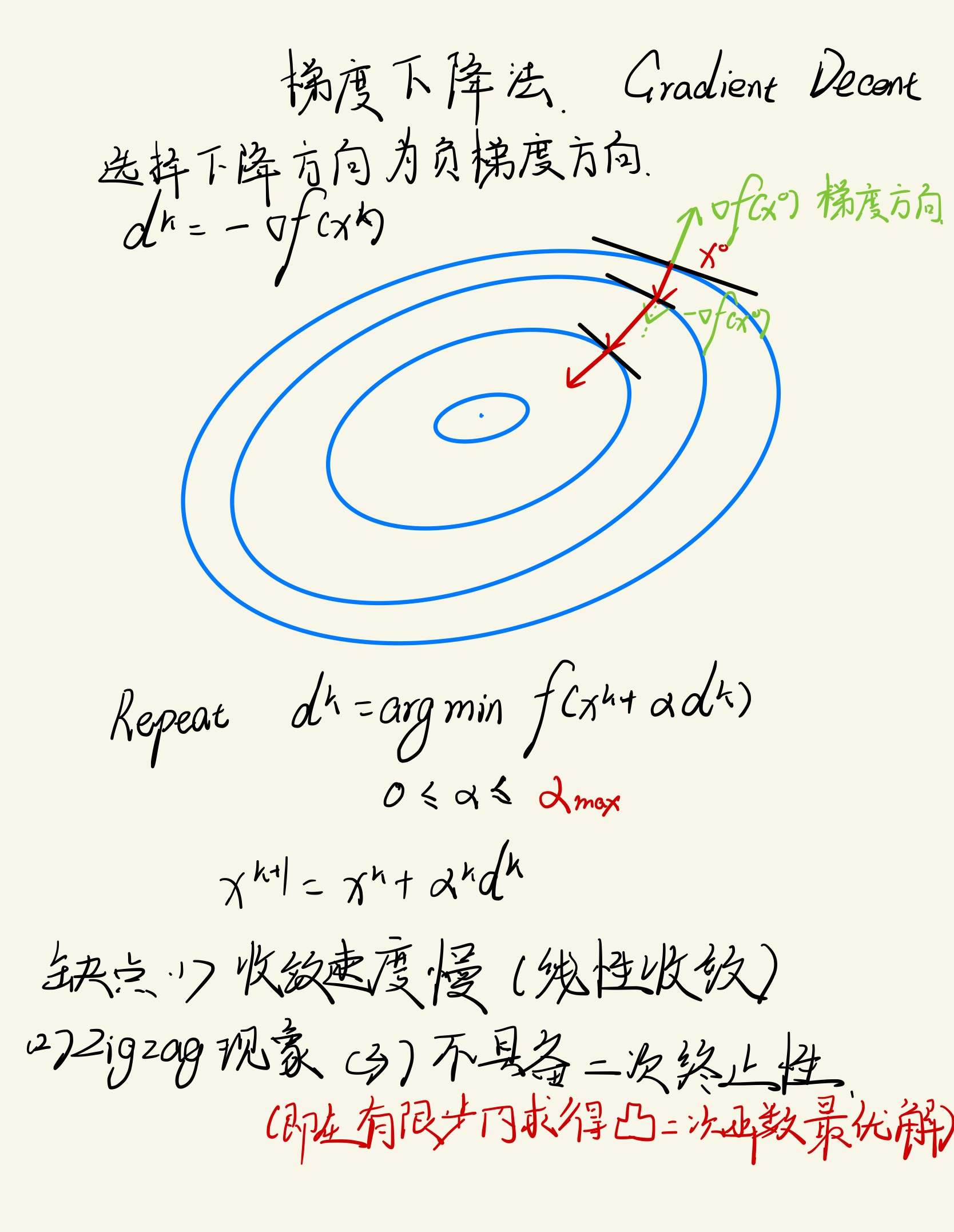分析算法收敛性 - 精确线搜索exact line search

∀

x

∈

d

o

m

f

,

M

I

⪰

∇

2

f

(

x

)

⪰

m

I

\forall x \in d o m f, M I \succeq \nabla^{2} f(x) \succeq m I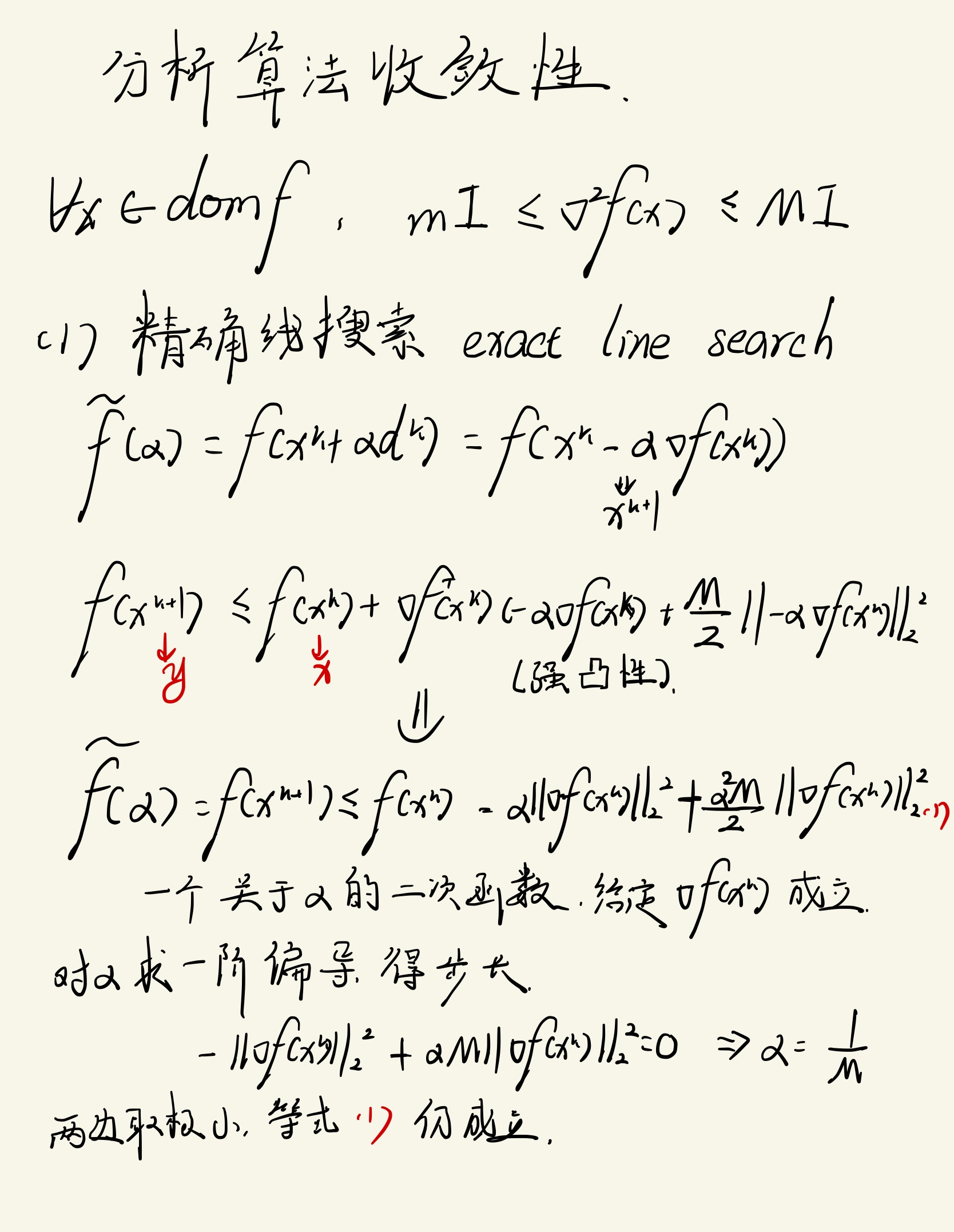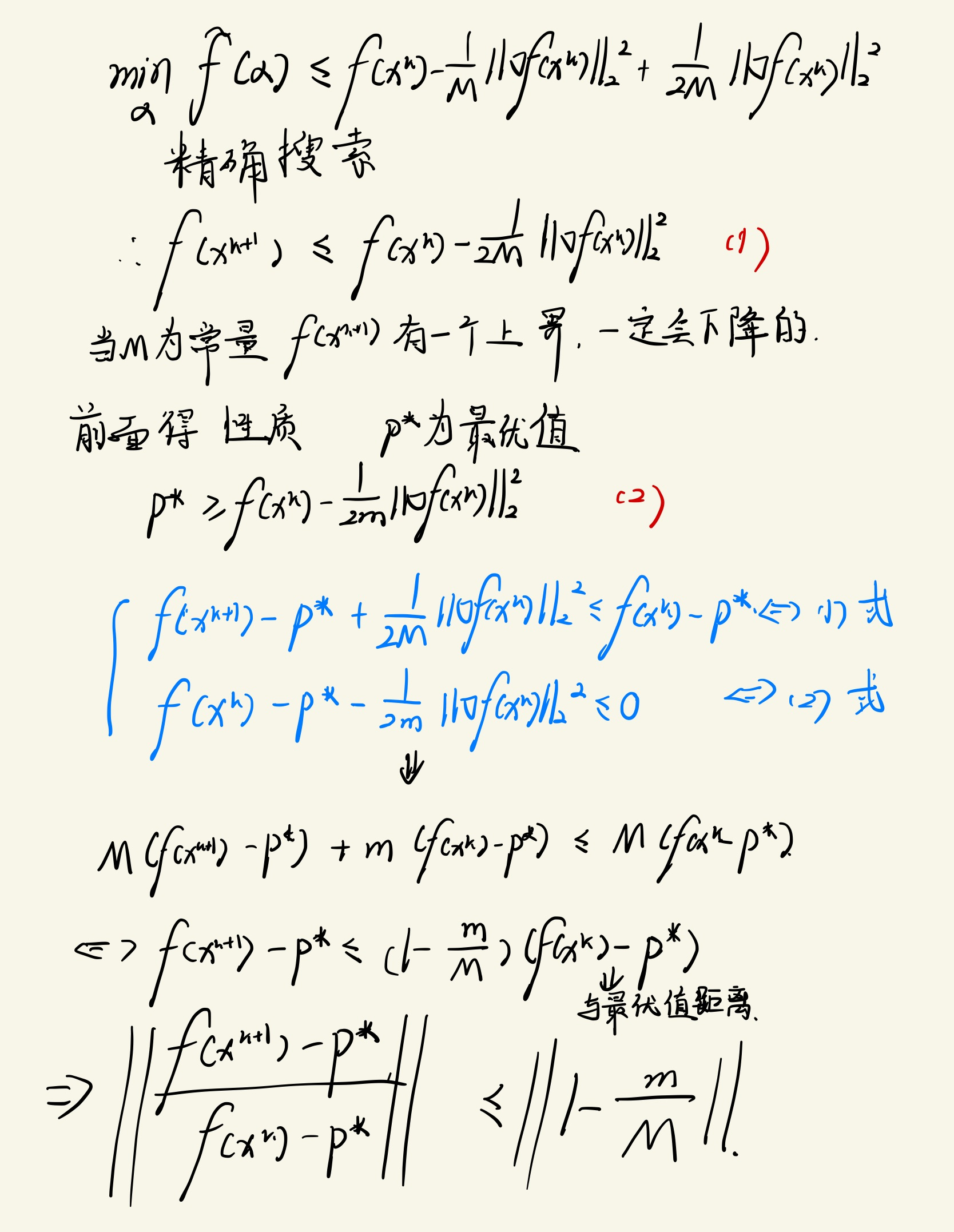分析算法收敛性 - 非精确线搜索Inexact line search(Amijo Rule)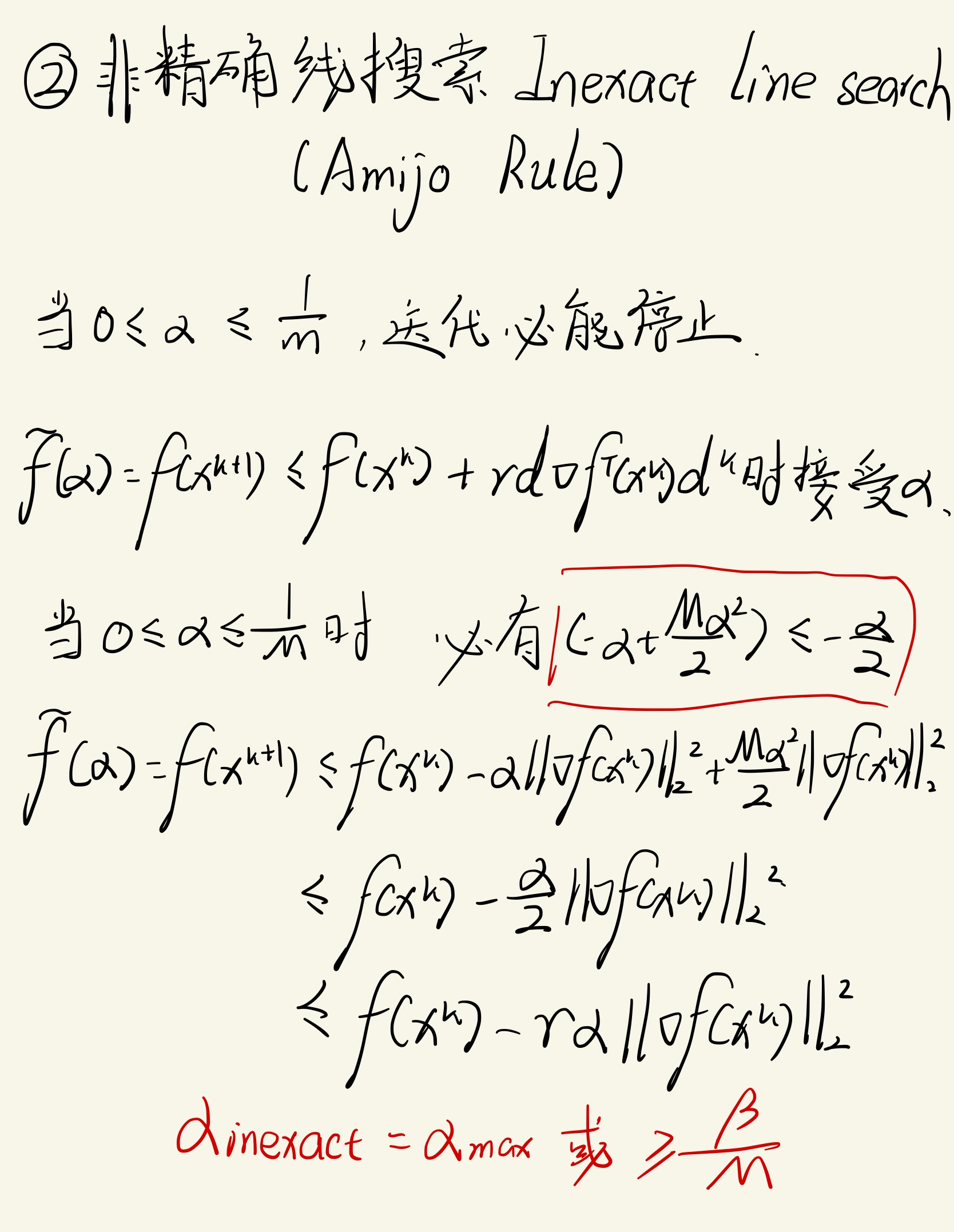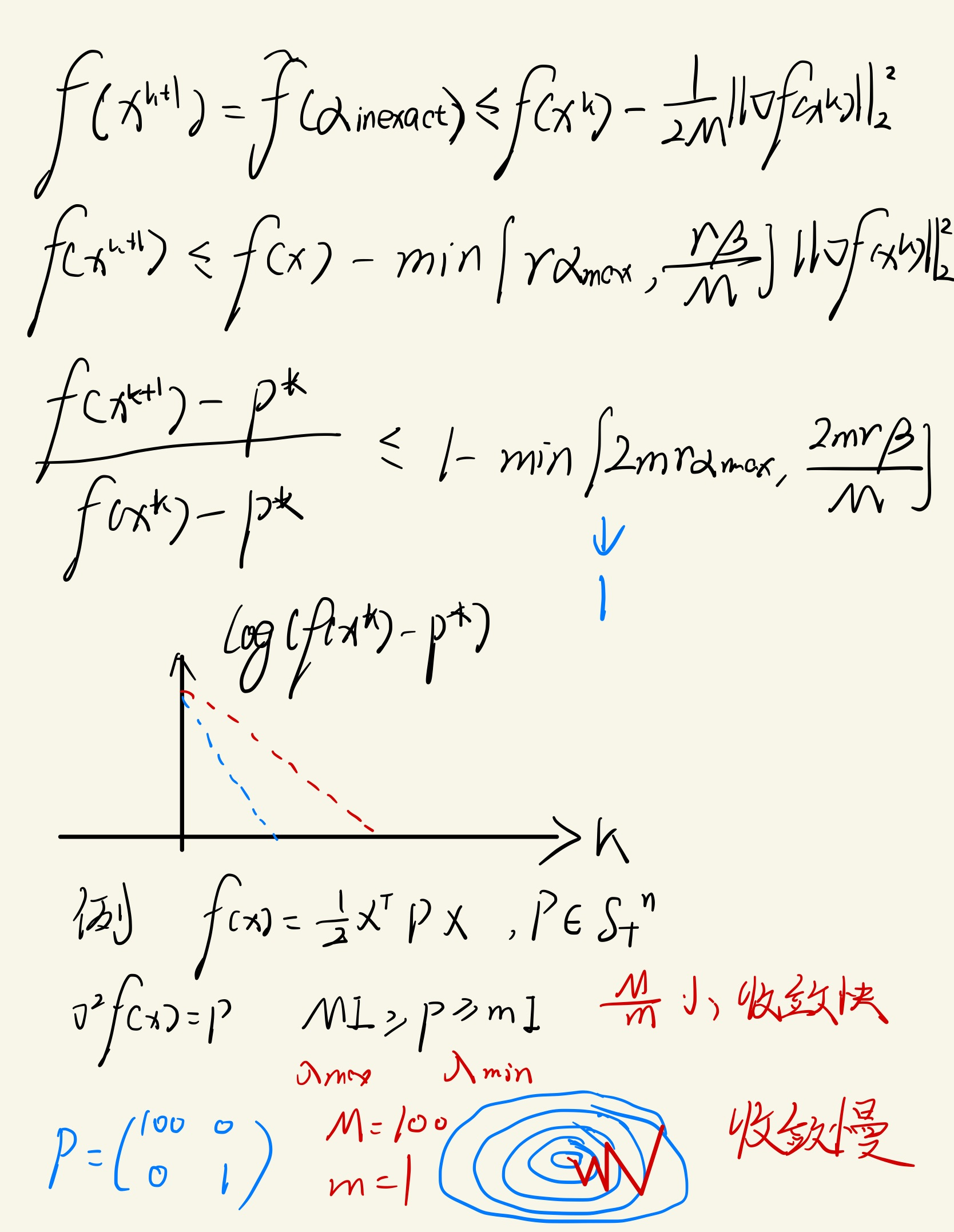展开全文最优化方法 数值优化
• 警示传播算法（WP）作为信息传播算法的基本模型，分析WP算法的收敛性对于研究其他信息传播算法的收敛性具有重要意义，分析了WP算法收敛性与结构熵之间的关系，给出WP算法收敛的判定条件。通过实验分析，该方法有效...
• 最近在看资料时，遇到了这样的说法“某某算法具有收敛快的优点”，于是便有点疑惑：收敛不是函数或者数列才有的概念吗？用到算法上是代表什么意思呢？...知道了算法收敛性的含义，再来理解算法收敛速度就比较容易了..
最近在看资料时，遇到了这样的说法“某某算法具有收敛快的优点”，于是便有点疑惑：收敛不是函数或者数列才有的概念吗？用到算法上是代表什么意思呢？遂查阅资料，将一点理解记录如下。

算法收敛性
算法的收敛性就是指某个算法能否在迭代时间趋于无穷的假设下，最终找到问题的全局最优解。这里有一点要明确：算法收敛性是迭代法中的一个概念，所以主要针对跟迭代相关的算法，如进化算法。对于能够一次求解的直接法，就不在算法收敛的讨论范围之内了。

算法收敛速度
知道了算法收敛性的含义，再来理解算法收敛速度就比较容易了。百度百科对算法收敛速度是这样解释的：
在数值分析中, 一个收敛序列向其极限逼近的速度称为收敛速度(Rate of convergence). 该概念多用于最优化算法中; 其被定义为一个迭代序列向其局部最优值逼近 (假设计算过程收敛, 并能达到最优值) 的速度, 是评价一个迭代法于该问题中发挥的性能的一个重要指针。
说白了，算法收敛速度就是指算法需要经过多少次迭代才能得到最优解。很明显，有些算法的收敛性好，有些算法的收敛性差，所谓收敛性好就是收敛得快，快速收敛的意义就是使用较少的迭代次数便可得到相对精确的值，或者说是在允许的时间内得到满意结果。因此，能以较快速度收敛于最优解的算法，才能称得上一个好算法。

最后再贴一段关于收敛性的论述来帮助理解：
仅仅知道算法是收敛的还远远不够，收敛性的结论是建立在无穷迭代时间基础上的，而实际应用中的计算迭代时间是有限的。收敛性研究只能回答进化算法在迭代无穷次后最终会不会找到全局最优解，而不能回答算法实际究竟要花多长时间（迭代多少次）才能找到最优解，很难在实践中用于指导算法设计和改进。

关于如何证明一个算法的收敛性以及对收敛速度的分析，有兴趣的可以看以下资料：
1.https://xueshu.baidu.com/usercenter/paper/show?paperid=f70e65d2b08ddb59a2602e9ff1593033&site=xueshu_se

参考资料：

几篇有关算法收敛性的文献分享：链接：https://pan.baidu.com/s/1QkyiKEzlFF7LF9JsaQOG7w      提取码：egef 
展开全文• 分析基于免疫响应原理的免疫进化算法流程和运行机制.根据免疫抗体群的状态转移过程,研究免疫...通过实验总结影响免疫进化算法收敛性的关键因素,为解空间较大及高维优化问题的免疫进化算法收敛性和性能分析提供可行方法.
• 论文引入鞅方法取代传统的马尔科夫链理论,研究保留精英遗传算法(EGA)的收敛条件和收敛速度.通过 把EGA的最大适应值函数过程...使用鞅方法分析 遗传算法收敛性具有独特的优势,成为分析遗传算法收敛性及其性能的新方法.
•研究论文
• 针对WP算法收敛性的研究，基于骨干集和后门集定义WP-可解公式，利用在G（n，3，m）模型和植入指派模型下证明WP算法的收敛性，给出算法收敛的充要条件。最后，通过在植入指派的公式产生模型上进行数值实验验证，结果...
• LMS自适应滤波算法,lms自适应滤波算法 收敛因子,C,C++源码
• LMS自适应滤波算法,lms自适应滤波算法 收敛因子,C,C++源码.rar
• 为了探讨WP(警示传播)算法的收敛性,给出了WP算法收敛的后门集。通过对此后门集中的变元赋值,可将布尔公式简化成其因子图为树型结构的子公式,WP算法在子公式上收敛。最后,设计了一个求解该后门集的随机算法,并分析了...
•研究论文
• 将多目标进化算法收敛到Pareto前沿的均匀分布表示研究论文
•粒子滤波
• 无限支撑面具级联算法收敛性的一个频域刻画，延卫军，李万设，级联算法在小波分析和信号处理中有着重要的作用. 本文从频域角度研究了无限支撑面具的级联算法的收敛性, 利用推移算子和一致可积�
• 网络结构熵对RGGs中分布式同步算法收敛速度的影响。研究论文
• 针对希尔伯特空间中的一般变分不等式,将其等价转化为变分包含问题.利用非精确邻近点算法将问题...在算子F是g-单调和算子g是同胚映射的条件下,得到非精确邻近点算法收敛于一般变分不等式的一个解,证明了解是唯一的.
• 论文研究-关于Tabu Swarch算法收敛性的研究.pdf,
• 针对免疫遗传算法收敛性质的研究非常缺乏，提出了利用随机过程理论和引入遗传吸收率、散射率参数进行分析的方法。通过数学建模证明了免疫遗传算法所形成的种群序列的强马尔可夫性，利用遗传吸收率和散射率的计算，...
• 考虑时滞因素的电力系统迭代辨识广域阻尼控制算法收敛性研究.pdf
• EM算法收敛性的推导
EM算法收敛性的推导
当第t次迭代开始时，将上一步的参数计算先验概率分布和条件概率分布以求出后验概率分布为

P(Z∣Y,θ(t))
$P(Z \mid Y,\theta^{(t)})$，带入下限函数B得

B(θ(t),θ)=∑zP(Z∣Y,θ(t))logP(X,Y∣θ)P(Z∣Y,θ(t))

B(\theta^{(t)},\theta)=\sum_z P(Z \mid Y,\theta^{(t)}) \log \frac{P(X,Y \mid \theta)}{P(Z \mid Y,\theta^{(t)})}似然函数L和下限函数B在

θ(t)
$\theta^{(t)}$这一点相等，如下

B(θ(t),θ(t))−L(θ(t))====∑zP(Z∣Y,θ(t))logP(X,Y∣θ(t))P(Z∣Y,θ(t))−logP(Y∣θ(t))∑zP(Z∣Y,θ(t))logP(X,Y∣θ(t))P(Z∣Y,θ(t))−∑zP(Z∣Y,θ(t))logP(Y∣θ(t))∑zP(Z∣Y,θ(t))logP(X,Y∣θ(t))P(Z∣Y,θ(t))P(Y∣θ(t))∑zP(Z∣Y,θ(t))log1=0

\begin{eqnarray*} B(\theta^{(t)},\theta^{(t)})-L(\theta^{(t)}) &=& \sum_z P(Z \mid Y,\theta^{(t)}) \log\frac{P(X,Y \mid \theta^{(t)})}{P(Z \mid Y,\theta^{(t)})} - \log P(Y\mid \theta^{(t)}) \\ &=& \sum_z P(Z \mid Y,\theta^{(t)}) \log\frac{P(X,Y \mid \theta^{(t)})}{P(Z \mid Y,\theta^{(t)})} - \sum_z P(Z \mid Y,\theta^{(t)})\log P(Y\mid \theta^{(t)}) \\ &=& \sum_z P(Z \mid Y,\theta^{(t)}) \log\frac{P(X,Y \mid \theta^{(t)})}{P(Z \mid Y,\theta^{(t)})P(Y\mid \theta^{(t)})} \\ &=& \sum_z P(Z \mid Y,\theta^{(t)}) \log1 = 0 \end{eqnarray*}
由于对

B(θ(t),θ)
$B(\theta^{(t)},\theta)$求极大值在

θ(t+1)
$\theta^{(t+1)}$取得，因此

B(θ(t),θ(t))≤B(θ(t),θ(t+1))

B(\theta^{(t)},\theta^{(t)}) \leq B(\theta^{(t)},\theta^{(t+1)})又由B是L的下限函数，因此

B(θ(t),θ(t+1))≤L(θ(t+1))

B(\theta^{(t)},\theta^{(t+1)}) \leq L(\theta^{(t+1)})综上，

L(θ(t+1))≥L(θ(t))

L(\theta^{(t+1)}) \geq L(\theta^{(t)})又似然函数一定小于1大于0，那么似然函数有界，函数单调又有界必收敛，似然函数的收敛性得证。

展开全文• 针对希尔伯特空间中的一般变分不等式,将其等价转化为变分包含问题.利用非精确邻近点算法将问题...在算子F是g-单调和算子g是同胚映射的条件下,得到非精确邻近点算法收敛于一般变分不等式的一个解,证明了解是唯一的.
• 标准粒子群优化算法收敛性能分析与参数选择，林川，冯全源，基于离散时间线性动态系统理论，推导了确定性标准粒子群优化（PSO）算法收敛，临界稳定与发散的充分必要条件，并用若干个粒子运动粒子群
• 但是对其性质的分析仍然不完善，针对以往文献对均值移动算法收敛性证明的错误和不足，根据柯西收敛定理严格证明了均值移动算法的收敛性；证明了基于任意核，两连续均值移动矢量的夹角都不大于90°。
• 算法 归一化很实用， LMS和归一化LMS算法收敛门限与步长的确定
• 应用马尔科夫链模型证明了遗传禁忌搜索算法是以概率1收敛到全局最优解的,并应用求解随机算法时间复杂度的方法,即求解算法的期望收敛时间,估算了该算法的时间复杂度,结果证明该算法的时间复杂度与所得解的多样性、...
• 但影响无功优化算法实际运行时的收敛性有两个因素：一是在优化问题可行域为空时，算法是否对不可行情况进行探测和处理；二是在求解非线性的无功优化问题时，是否对模型的准确性进行限定。首先，介绍了评价和选择一个...
• ## Kmeans 算法收敛

千次阅读 2018-12-10 20:19:47
我们要知道，其实K-means是一种为了简化计算以及加快收敛速度的近似算法，为什么？你看算法在距离上的定义 D=||xk−μ^i||2 是不是很熟悉呢，对的就是欧式距离 凭什么要用欧式距离？用别的距离不...
转载自https://ask.julyedu.com/question/143?notification_id=10011
仅mark，侵删

下面我们来聊一下K-means。 我们要知道，其实K-means是一种为了简化计算以及加快收敛速度的近似算法，为什么？你看算法在距离上的定义
D=||xk−μ^i||2
是不是很熟悉呢，对的就是欧式距离 凭什么要用欧式距离？用别的距离不行？（K-means：我乐意，不服你来打我啊） 我们下面来聊一聊用别的距离会怎么样，为了方便起见，我们就用跟欧式距离很像的马氏距离好了
D=(xk−μ^i)TΣ^−1i(xk−μ^i)
在此之前，我们要知道，聚类究竟是要做什么，聚类可以认为是这样的 1. 样本的类别c需要知道，比如你拿到一堆没有标签的数据，他们是手写字母，那么类别就应该是26（你要是跟我扯大写小写一共52个类别就是不客观，你就是来砸场的哼）。但是如果这些数据是类别不明确的，你根本就没办法知道有多少个类别，或者本来就没有类别的概念，那么这个c就取决你了，你可以凭直觉（只要你boss不踢你滚蛋），凭经验啥的来决定c取多少，但不管怎样，这个c是一定知道的。 2. 每个类别的先验概率P（w_i）也是知道的，这个如果你其他参数都定了，那么这个参数就能算出来。 3. 样本条件概率的数学形式是以知的，即
p(x|wj,θj),j=1,⋯,c
是知道的，可能这个形式是你本来就知道这个模型是这样，或者你猜这个模型是这样（俗称：蒙的），不管怎样，我说你知道你就是知道别抵抗！ 4.参数是未知的，即我们不知道以下参数
θ1,θ2,⋯,θc
的具体取值是什么，但是他们长什么样是知道的（因为模型是知道的） 所以聚类任务就可以看做是，我知道模型，知道类别，唯独不知道类别标签，所以有监督学习与无监督学习是很像的（其实这两个的界限本来就很模糊），如果你是学自动控制的，你会发现：这TM不就是系统辨识吗？！ 好像扯远了，回到我们的k-means上来，k-means实际上是一种对数似然函数空间的随机梯度法，这个一会我们我们再回来聊，我们先来解决一个问题：我们刚还扯着聚类，怎么就突然扯到似然函数了？？？ 根据我们之前关于聚类问题的定义，我们不难得出从一个混合密度中采样到一个样本的概率为
p(x|θ)=∑j=1cp(x|wj,θj)P(wj)
（友情提示：你可以认为上面的式子就是，对于某个样本x，他属于A类的概率，B类的概率.....所有类别的概率之和） 如果说这时候参数θ的数值知道了，那么整个问题就解决了，但是我们不知道，所以才会有后面的一大堆问题 不知道怎么办呢？回想我们的概率论，参数不知道，那就拿极大似然去撸他咯 友情提示：如果你忘了极大似然的含义，那么你可以理解为极大似然就是，我观察到这个现象，那么我就要让参数使得我观察到这个现象出现的概率最大。 举个栗子，现在有两个人，某位低调的网红小王，以及我。还有一个参数---某位地产大亨健林先生，健林先生这个参数只能取两个值：小王的父亲、或是我的父亲，但是这个取值我们不知道。好了背景介绍完毕，现在发生了一件事，我和小王同时追一名女生，结果女生对我说“你是个好人”。那么问题来了，参数取值为什么？当然是“小王的父亲”啊，为什么？因为这个取值使得我被发好人卡的概率最大。这就是极大似然的中心思想。 好了，回到原来问题上的讨论，对于训练集合D，我们有
p(D|θ)=∏k=1np(xk|θ)
当然了，我们往往都是撸对数似然的，因为连加要比连乘容易处理，所以我们有
l=∑k=1np(xk|θ)
代入混合概率密度并求导，得到
∇θil=∑k=1n1p(xk|θ)∇θi[∑j=1cp(x|wj,θj)P(wj)]
假设我们的参数θ1, θ2....是独立的，那么引入后验概率
p(wi|xk,θ)=p(x|wj,θj)P(wj)p(xk|θ)
于是我们可以将似然函数的梯度改写成：
∇θil=∑k=1nP(wi|xk,θ)∇θilnp(xk|wi,θi)
友情提示：这个可以反推回去的，自己试着推一下吧：D 在数学推导上，接下来就是令梯度为0，然后求解参数blahblah

好了，回到我们之前的话题，我们之前讲到似然函数，大家有没有发现，上面的推导跟有监督学习的推导是一模一样的！！！！！ 是的，上面的就是有监督学习的推导=。= 诶诶诶？？同学！别打脸，我明天还要出门见人的 为什么说上面的是有监督的推导？因为在这里我们假定我们是知道先验概率P（w_i）的，也就是说，我们知道各个类别的出现概率的，问题就出现了，我们现在是无监督的啊，一开始就没给标签你你哪来的先验概率？ 接下来我们就将上面的东西推广到P（w_i）也未知的情况，下面问题就变成了要求一组参数θ以及一组P（w）使得似然最大，且这组P（w）还要满足概率三大公理的前两条：
P(wi)≥0,i=1,⋯,c,           ∑i=1cP(wi)=1
如果我们分别定义P（w_i）和θ的最大似然估计为：
P^(wi),   θ^i
那么我们有
P^(wi)=1n∑k=1nP^(wi|xk,θ^)
以及
∑k=1nP^(wi|xk,θ^)∇θilnp(xk|wi,θ^i)=0
其中
P^(wi|xk,θ^)=p(xk|wi,θ^i)P^(wi)∑cj=1p(xk|wj,θ^j)P^(wj)
解释一下上面两个个式子 第一个式子：类别概率的最大似然估计是每个样本估计之和然后求平均，体现贝叶斯的思想 第二个式子：这是最大似然估计（令导数为0），体现最大似然思想 终于进入到聚类的领域了，上面这两个式子，第一个，你可以理解为k-means的第一阶段；第二个，你可以理解为k-means的第二阶段。事实上，k-means和EM算法是很像的，非常非常像，不信你仔细想想EM是不是也在做同样的事情？只不过表达换了一下而已。 好了，回到我之前说的那句话“k-means实际上是一种对数似然函数空间的随机梯度法”，现在大家应该已经知道为什么会出现似然空间这个说法了，下面我们依然不打算直接讲k-means，我们先来看下面这个图上面三条曲线，其中实线是真实的概率密度，而两条虚线是分别是两种估计，事实上我们也不难看出，A那条曲线要比B的更准确，但是就能说A更好吗？如果我们开了上帝视角当然能这么说，但实际中我们不知道真实的密度是怎么样的，所以A和B两条曲线差不多，没有那个更好。此外，如果我们一开始类别设置错了，我们设置成了3个类别，那么得到的曲线又不一样了，所以，我们对于类别的设置也对算法起到很重要的作用。 但事实上，哪怕类别C设定准确了，也不一定能收敛到全局最优点，为什么？看下面这张马鞍面图中的红线是迭代过程中最大似然的轨迹，如果我们初始化的位置比较好，那么可以上升到最顶点，如果不好呢？那就有可能收敛到鞍点，所以我们的观点是：多在几个不同的初始点试几次，选最好的来用。 好了回到k-means上来。在上面那个模型中，我们知道他是高斯模型，所以我们就使用马氏距离，所以对于概率
P^(wi|xk,θ^)=p(xk|wi,θ^i)P^(wi)∑cj=1p(xk|wj,θ^j)P^(wj)
我们经过推导是可以证明它随着
(xk−μ^i)TΣ^−1i(xk−μ^i)
的减小而增大，关于这个证明，我实在是不想敲公式了。。。原谅我。。。。。。 但如果我们换成欧式距离呢？也就是
||xk−μ^i||2
然后通过欧氏距离找到中心，并对概率进行简化
P^(wi|xk,θ^)≈1,若i=m

P^(wi|xk,θ^)≈0,否则
然后我们就得到了K-means，所以“k-means实际上是一种对数似然函数空间的随机梯度法”这种说法明白了吗？ 依然是上面的曲面，我们看下它的等高线图，在k-means中，他的上升轨迹如下：从不同的起始点，我们可以看到k-means将收敛到不同的点，大部分情况下是到全局最后点，但也有收敛到鞍点的情况。 聚类，其实是比较主观的事，比如下面这个图，我既可以说它可以分为两类，也可以说它可以分为3类，所以这种事，你开心就好此外，聚类得到的结果也不一定是正确的，比如下面这张图，上面部分是聚类的结果，下面部分才是其真实情况K-means简单粗暴有成效，是个挺有效果的算法，但不意味着它总能奏效，比如下面这种情况：如果我们继续使用k-means聚类：很显然这时候k-means就不在奏效了，对此我们有谱聚类这种算法，那个太跑题了，而且我也不太懂那块，比如下面是对上图谱聚类的结果这其实不能说K-means就一无是处，事实上，在机器学习里，每个算法都是有用处的，只有适不适合，没有谁比谁本质上更好，比如用logistic regression就能工作得很好的工作你就没必要搬卷积神经网络这种大炮出来，而且这座大炮还不一定工作得比LR好 楼主对不起=。= 我扯了半天也没回答你的问题 对于你的问题，我觉得，可以多换几个初始值试试，关于收敛则准则有两种，一种是判断中心移动（如果你的中心已经不怎么变化了说明收敛了阿，你可以将误差记录下来看看误差下降情况），另外一种是根据迭代次数，k-means我印象中是收敛的吧=。=
展开全文聚类...